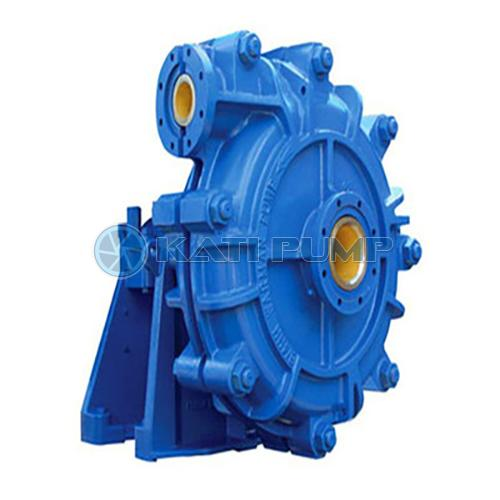# The selection steps of slurry pump are as follows

The selection steps of slurry pump are as follows

1. The critical velocity VL was calculated according to slurry parameters s, D and CW.

2. According to the given flow and pipeline conditions, the head loss of clean water pipeline is calculated, and the characteristic curve of clean water pipeline is drawn.

3. The characteristic curve of slurry pipeline is drawn, and the water head under the given flow rate is calculated.

4. Calculate the clear water head and increase the head by 10% to get the required head.

5. Determine the type of slurry pump according to slurry characteristics.

6. According to the given flow rate and the clear water head of the pump, the specification of the pump should be selected. When a pump can not meet the head requirements, series connection should be considered. For the series pump, the working pressure and bearing life of the pump should be calculated, and whether it can meet the requirements should be judged.

7. Draw the characteristic curve of clean water and slurry pipeline in the performance curve of the selected pump, get the operating point of the pump, and find out the value of N, NPSHr and η of the pump.

8. Npsha was calculated. To judge whether the pump is operating without cavitation, the flow at the working point after increasing the head margin should be considered in the calculation (e.g. npsha < NPSHr + 0.3, the installation condition of the pump inlet can be changed or the pump type can be re selected).

9. Calculate the slurry shaft power of the pump, check whether the shaft power is less than the bracket power of the selected pump, and select the standard motor (the head should be the head after the margin is increased in the calculation).

10. According to the speed and power to determine the transmission type, installation type and shaft seal type.

11. The outlet pressure of the packing shaft seal is calculated to determine the water volume and water pressure of the packing shaft seal.

Before the calculation, we need to understand the problems of slurry pump selection.

Calculation formula of slurry pump selection

Now the formula of slurry pump selection in various industry standards is listed, and the symbols in the formula are unified.

1) Typical slurry process

Pipeline characteristics:

Clear water HF = Δ H + (1 + ξ) (V ^ 2) / (2G)

Slurry HMO = ΔΗ + 0.72ko (VL ^ 2) + 0.58ko (V ^ 2)

Pump characteristics:

Slurry HM = ΔΗ + 0.72ko (VL ^ 2) + 0.58ko (V ^ 2)

Clear water HS = HS * HR

2) Coal preparation plant method 1

Pipeline characteristics:

Clear water HF = Δ H + IL + 2

Slurry HMO = ΔΗ + IML + 2

Pump characteristics:

Slurry HM = ΔΗ + IML + 2

Clear water HS = H / km

3) Ash removal calculation method

Pipeline characteristics:

Clear water HF = Δ H + 1.05il

Slurry HMO = ΔΗ γ m + 1.05iml

Pump characteristics

Slurry HM = 1.1hmo

Clear water HS = HS * γ m * km

4) Tailing calculation method

Pipeline characteristics:

Clear water HF = Δ H + IL + Σ hi

Slurry HMO = ΔΗ γ m + IML + Σ hi

Pump characteristics:

Slurry HM = ΔΗ γ m + IML + Σ hi

Clear water HS = HS * γ m * km * KH

5) Filling mining method

Pipeline characteristics:

Clear water HF = Δ H + 1.05il + ∑ hi

Slurry HMO = ΔΗ γ m + IML + Σ hi

Pump characteristics:

Slurry HM = ΔΗ γ m + IML + Σ hi

Clear water HS = HS * km * KH

6) Metallurgical Mining Law

Pipeline characteristics:

Clear water HF = Δ H + IL + Σ hi

Slurry HMO = ΔΗ γ m + IML + Σ hi

Pump characteristics:

Slurry HM = ΔΗ γ m + IML + Σ hi

Clear water HS = HS * γ m * KH

The symbol and meaning in the formula

The water head of HF, HMO, HM and HS pipeline and the head of slurry, the head of slurry and the head of clean water of pump body;

L long pipeline;

i. Im the friction resistance coefficient between water and slurry;

Kh=1-0.25Cw

γ m slurry specific gravity;

Ko = H / (V ^ 2), and the ratio of water head to velocity square is calculated.

VL critical settling velocity.

Km = hm / (VM ^ 2) slurry to calculate the ratio of pipeline head to velocity square.Name: KATImachinery

Mobile：+86031180632344

Tel：+86031180632344

Email：info@katimachinery.com

Add：306-1 Zhongchuang building 5,No.251 Yucai street, Shijiazhuang China

﻿
Scan the qr codeClose